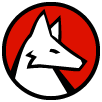Wolfram 语言：快速编程入门关联观看视频

Java 程序员备注：

Wolfram 语言的 Associations 与 Java 中的 MapHashMap类似，但有着更好的语法可读性和更灵活的使用方法.

Python 程序员备注：

Associations 在 Wolfram 语言中与 Python 的字典作用类似. 使用 Association，程序员可以用高效的查找和更新将键值相关联，即使有上百万种类元素，同时还需要在不使用例如 Python 的 OrderedDict 的特定的数据结构来保持这些元素的插入顺序.

（用 `->` 输入 →）

In:=1`<|"a" -> x, "b" -> y|>`
Out=1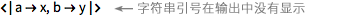（关联中的键经常使用字符串，但它不是必需的.）

In:=2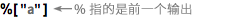`%["a"]`
Out=2In:=1`{#b, 1+#b} & [<|"a"->x, "b" -> y |>]`
Out=1In:=1`<|"a" -> x, "b" -> {5, 6}|>[["b", 1]]`
Out=1In:=1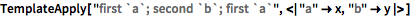`TemplateApply["first `a`; second `b`; first `a`", <|"a" -> x, "b" -> y|>]`
Out=1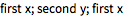x=<|"cat" `→` <|"vet" `→` "Eve", "age" `→` 5|>, "dog" `→` <|"vet" `→` "Abe", "age" `→` 4|>|> 中提取猫的年龄？

meal=<|"appetizer" `→` "nachos", "salad" `→` "spinach", "dessert" `→` "chocolate"|> 中创建带有 "appetizer" 和 "dessert" 的字符串？